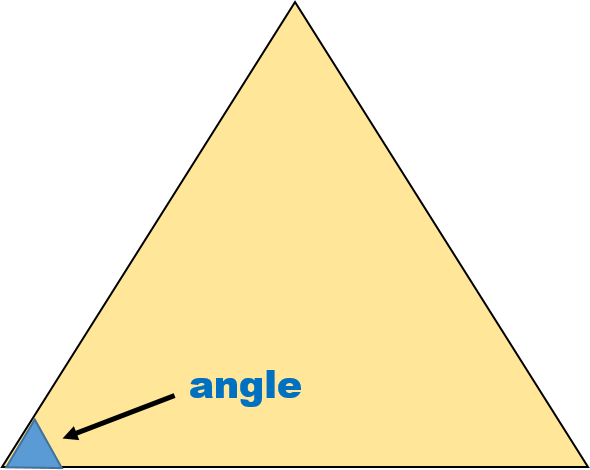1
visibility

Which of the following shapes has fewer angles?

• A• BThe correct answer is.

Please Note:
An angle measures the amount if turn. It is formed where two sides of a shape meet.

See example:We count the total number of angles.

There are 3 angles.

Solution:

Consider the shape below:We count the total number of angles.

There are 3 angles.

Consider the shape below:We count the total number of angles.

There are 4 angles.

Since, 3 < 4

So,has fewer angles.